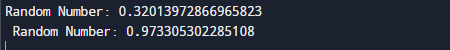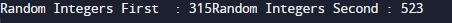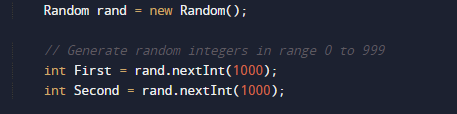# Java Program to generate Random Number

In this tutorial you will learn about the Java Program to generate Random Number and its application with practical example.

In this tutorial, we will learn to create a Java Program to generate Random Number using Java programming.

## Prerequisites

Before starting with this tutorial we assume that you are best aware of the following Java programming topics:

• Java Operators.
• Basic Input and Output function in Java.
• Class and Object in Java.
• Basic Java programming.
• If-else statements in Java.
• For loop in Java.

## What is Random Number?

Random numbers use choose a random in a large set of numbers and selects a number using the mathematical algorithm permutation and combination.

## Java Program to generate Random Number

In this program we would find a random number using builtin function in java .first of al we initiate required variables and function and then we will print random  on screen using builtin in function .let have a look at the code

Printing Random value using Math.random() function ;

## Outputin the above program we are printing random number using random.math() Built in function().this is how Math.random() function work.

Printing Random value using Java.util.Random class.

## Outputin the above program we are printing random number Java.util.Random class.
first of all we create an instance of this class and then invoke methods such as nextInt() to generate random number.this is how random class works.

There is one another method to generate random number using java.util.concurrent.ThreadLocalRandom class with the help of this class we also generate random number in a java program.

In this tutorial we have learn about the Java Program to generate Random Number and its application with practical example. I hope you will like this tutorial.